# Chemical Bonding

### Noble Gases

An atom is the smallest particle of an element that retains its chemical properties. Only six elements, known as noble gases are able to exist naturally as single unbounded atoms:

• Helium ($He$)
• Neon ($Ne$)
• Argon ($Ar$)
• Krypton ($Kr$)
• Xenon ($Xe$)
• Radon ($Rn$)

In the atoms of a noble gas:

• All electrons are paired with opposite spins
• The outer shell is full and is therefore extremely stable and unreactive.

### Octet Rule

The tendency to acquire a noble gas configuration is known as the Octet Rule.

## Bonding

Elements not in group 0 bond with other atoms in order to gain a full outer shell. The type of bonding that occurs depends on the atoms involved in the bond:

• Ionic bonding
This occurs in compounds consisting of non-metals and metals. In ionic bonding, electrons are transferred from the metal to the non-metal which forms oppositely charged ions.

• Covalent bonding
This occurs in compounds consisting of two non-metals. In covalent bonding, electrons are shared between atoms.

• Metallic bonding
This occurs in metals. In metallic bonding electrons are shared between all ions.

## Ionic Bonding

Ionic bonds are formed between non metals and metals. They are formed when electrons are transferred from the metal atom to the non metal atom. This creates two oppositely charged ions which are bonded by an electrostatic attraction. An ionic bond is an electrostatic attraction between two oppositely charged ions.

### Dot-cross diagrams

In order to show these bonds diagrammatically, a dot-cross diagram can be used. In these diagrams, a dot is used to show the electrons of one element and crosses for the electrons of the other.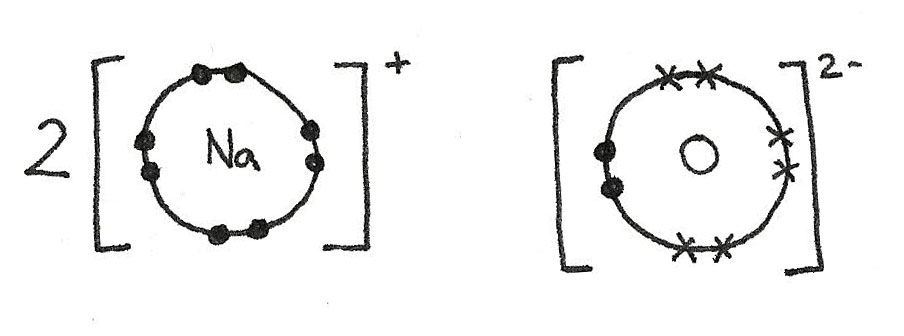The sodium atoms have each lost an electron in order to gain a full outer shell. This results in a positive charge.

\eqalign{ 2Na &\rightarrow 2Na^{+} + 2e^{-} \\ 1s^{2}\,2s^{2}\,2p^{6}\,3s^{1} &\rightarrow 1s^{2}\,2s^{2}\,2p^{6}}

The oxygen atom has gained two electrons to form a full outer shell. This has given the oxygen atom a 2- negative charge.

\eqalign{ O + 2e^{-} &\rightarrow O^{2-} \\ 1s^{2}\,2s^{2}\,2p^{4} &\rightarrow 1s^{2}\,2s^{2}\,2p^{6}}

### Giant Ionic Lattices

In the above example, only one bond is shown however in reality many ions are surrounded by many oppositely charged ions. These ions attract in all directions forming a giant ionic lattice.

A giant ionic lattice is a three dimensional structure of oppositely charged ions held together by strong ionic bonds.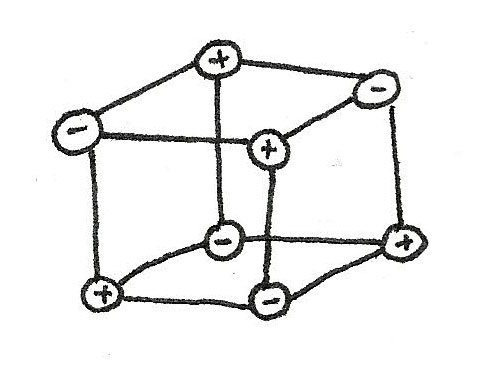## Ions and The Periodic Table

The charge of an ion of an element can be predicted from the position on the periodic table. The number of electrons in the outer shell can be found from the group the element is in. For example, lithium ($Li$):

• Is in group 1 therefore has one electron in its outer shell.
• In order to gain a full outer shell, it must lose one electron.
• A lithium ion ($Li^{+}$) must have a 1+ charge.

Some of the noticeable patterns are shown below:

• Atoms of metals in group 1-3 lose electrons, forming positive ions.
• Atoms of non metals gain electrons, forming negative ions.
• Atoms of $Be$, $B$, $C$ and $Si$ do not normally form ions as too much energy is needed to transfer the outer shell electrons to form ions.

### Molecular Ions

Groups of covalently bonded atoms can also lose or gain electrons to form ions. These are called molecular ions:

1+ 1-
Ammonium ($NH_{4}^{\kern{8px}+}$) Hydroxide ($OH^{-}$)
Nitrate ($NO_{3}^{\kern{8px}-}$)
Nitrite ($NO_{2}^{\kern{8px}-}$)
Hydrogencarbonate ($HCO_{3}^{\kern{8px}-}$)
2- 3-
Carbonate ($CO_{3}^{\kern{8px}2-}$) Phosphate ($PO_{4}^{\kern{8px}3-}$)
Sulfate ($SO_{4}^{\kern{8px}2-}$)
Sulfite ($SO_{3}^{\kern{8px}2-}$)
Dichromate ($Cr_{2}O_{7}^{\kern{8px}2-}$)

### Predicting Ionic Formulae

Although an ionic compound is made up of charged ions, the overall charge of a compound is 0. This means that ionic formulae can be predicted by looking at the charges.

### Worked Example

For calcium chloride, the overall charge must be zero. It is known that it is comprised on $Ca^{2+}$ ions and $Cl^{-}$ ions. For the charge to add up to zero there must therefore be two chlorine atoms and one calcium atom:

$$CaCl_{2}$$

## Covalent Bonds

Compounds containing two non metals form covalent bonds between atoms. Unlike ionic bonds where electrons are transferred, in a covalent bond, electrons are shared between two atoms. Covalent bonds can be shown using dot and cross diagrams: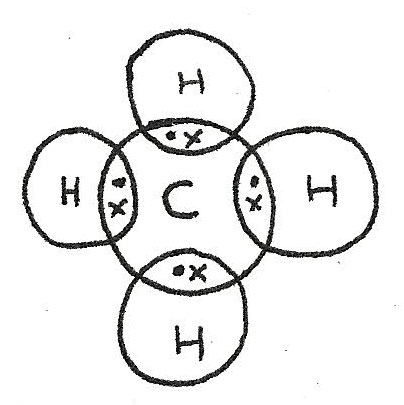In order to form a full outer shell, the carbon atom bonds with four hydrogen atoms. The number of bonds that an atom makes depends on the number of electrons in the outer shell.

#### Multiple Covalent Bonds

In some compounds, double bonds are formed which means that more than one pair of electrons are shared together.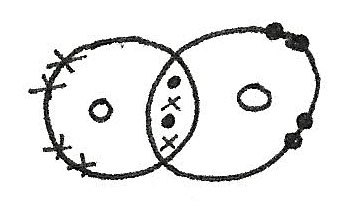### Lone Pairs

Lone pairs are pairs of electrons that are not used for bonding. These lone pairs form concentrated regions of negative charge which can affect the electronegativity of the compound and the shape.

### Dative Covalent Bonds

In dative covalent bonds, one of the bonded atoms supplies both of the shared electrons.

### Worked Example

An ammonium ion, $NH_{4}^{+}$, has three covalent bonds and one dative covalent bond. This is due to formation from $NH_{3}$ and $H^{+}$.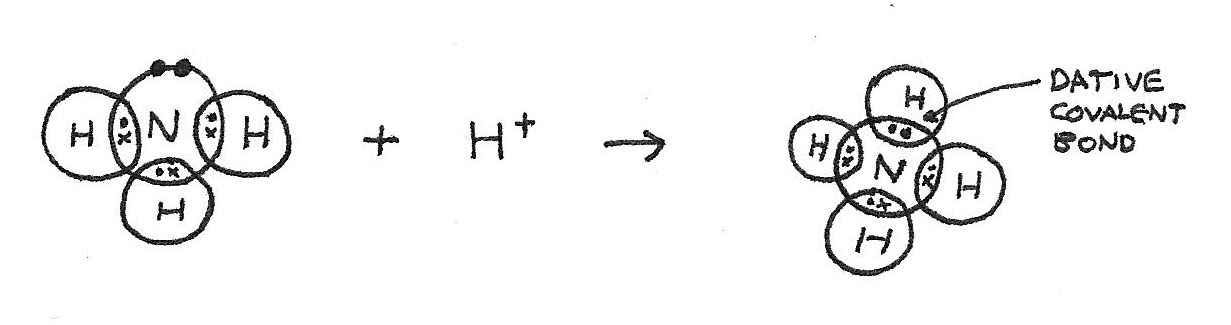1. In $NH^{3}$, there is a lone pair of electrons.
2. In the formation of $NH_{4}^{+}$, the lone pair provides both shared electrons.
3. Bonding with the $H^{+}$ means that the $NH_{4}^{+}$ ion has a positive charge of $+1$.

### Number of covalent bonds

When covalent bonds form, unpaired electrons often pair up so that the bonding atoms obtain a noble gas configuration, obeying the octet rule. However, this is not always possible:

• There may not be enough electrons to reach an octet. An example of this is in $BF_{3}$.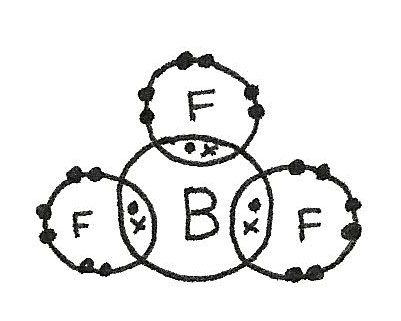• More than four electrons may pair up in bonding which is called the expansion of the octet. An example is $SF_{6}$.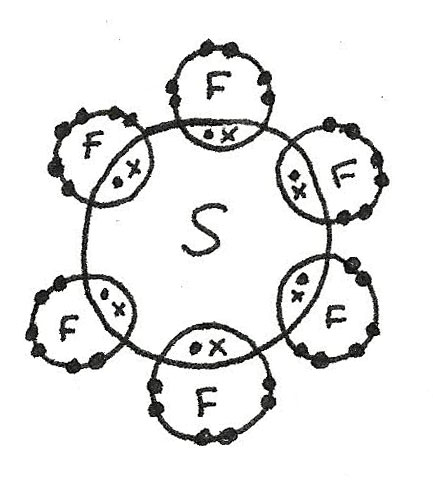## Shapes of Molecules

The physical shape of a molecule is determined by the number of electron pairs in the outer shell surrounding the central atom. This is due to the repulsion of the negatively charged electrons. Equal repulsion means that the bonded pairs push as far away from each other as possible.

Some molecules are shown below:

• $BF_{3}$ has a trigonal planar shape as there are three bonded electron pairs around the central atom. This gives a bond angle of 120°.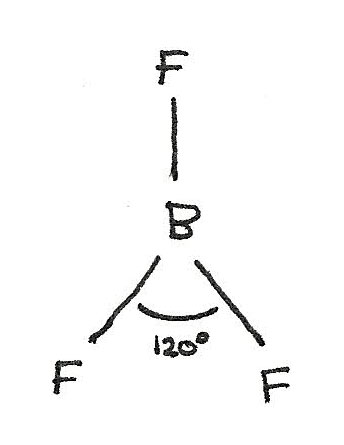• $CH_{4}$ has a tetrahedral shape as there are four bonded electron pairs. This gives a bond angle of 109.5°.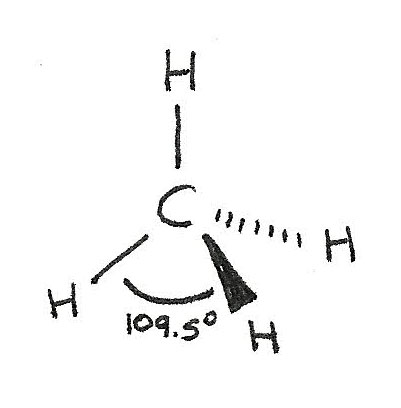• $SF_{6}$ has a octahedral shape as there are six bonded electron pairs. This gives a bond angle of 90°.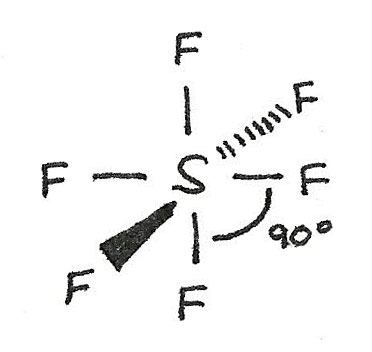Lone pair of electrons are more electron-dense than a bonded pair. This means that the repulsion is greater, which reduces the bond angle by around 2.5°.

#### Double Bonds

A double bond is classed as a single bonding region, meaning that the shape is the unchanged compared to a single bond.

Bonded Pairs Lone Pairs Name Angle
2 0 Linear 180°
2 2 Non-linear 104.5°
3 0 Trigonal planar 120°
3 1 Pyramidal 107°
4 0 Tetrahedral 109.5°
6 0 Octahedral 90°